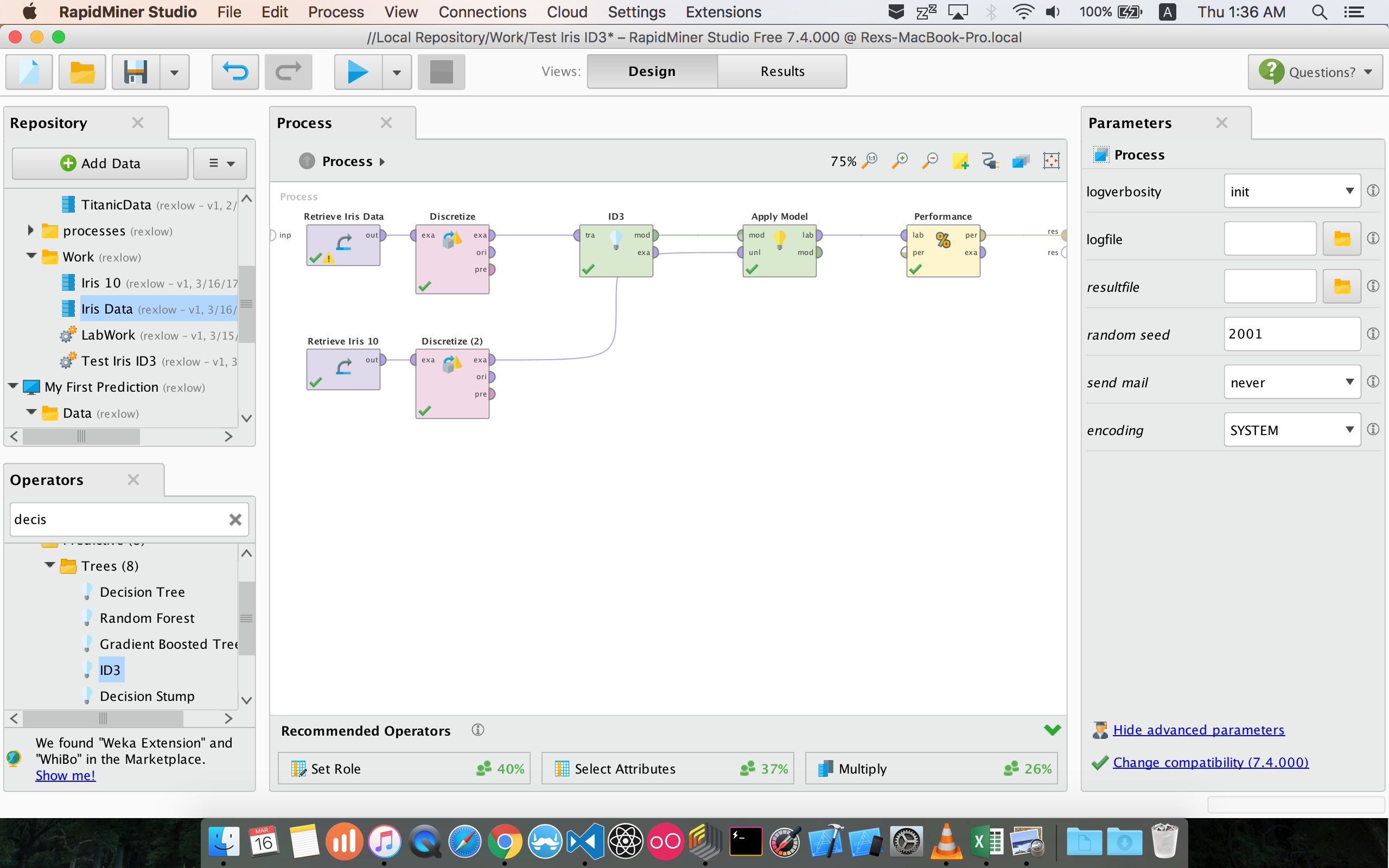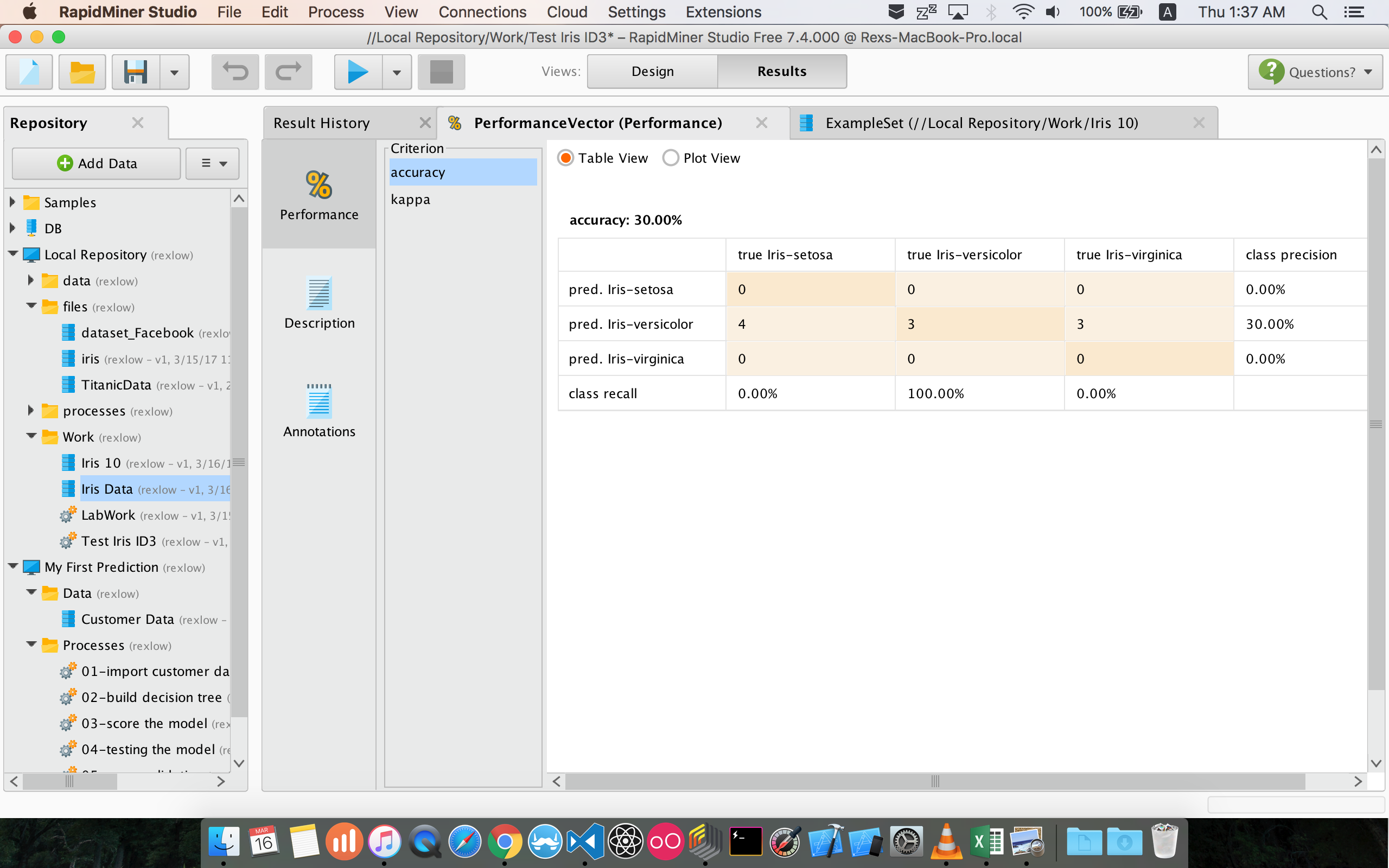# "Validate Accuracy with ID3 algorithm"

Member Posts: 11Contributor I
edited June 2019 in Help

Hi all, I am trying to validate a decision tree learning model but the result I got is just 30%, not sure where I did wrong.

I am using the UCI Iris data set and randomly selected 50 instances as my sample.

Then, I discretize the data with the operator "discretize by binning".

Next, dragged in "Apply Model" and "Performance".

Lastly, I randomly pick 10 instance from the original data set as the test data.

Here is my setup.This is the result i get.Apparently I get 30% accuracy as the result.

Does it mean my model is poorly design?

If my model is correct, how can I conclude the result? 30% is rather poor right?

Tagged:

• RapidMiner Certified Analyst, RapidMiner Certified Expert, Member Posts: 1,761Unicorn
`<?xml version="1.0" encoding="UTF-8"?><process version="7.4.000">  <context>    <input/>    <output/>    <macros/>  </context>  <operator activated="true" class="process" compatibility="7.4.000" expanded="true" name="Process">    <process expanded="true">      <operator activated="true" class="retrieve" compatibility="7.4.000" expanded="true" height="68" name="Retrieve Iris Data" width="90" x="45" y="34">        <parameter key="repository_entry" value="//Local Repository/Work/Iris Data"/>      </operator>      <operator activated="true" class="discretize_by_bins" compatibility="7.4.000" expanded="true" height="103" name="Discretize" width="90" x="179" y="34">        <parameter key="number_of_bins" value="3"/>      </operator>      <operator activated="true" class="concurrency:cross_validation" compatibility="7.4.000" expanded="true" height="145" name="Validation" width="90" x="380" y="34">        <parameter key="sampling_type" value="stratified sampling"/>        <process expanded="true">          <operator activated="true" class="id3" compatibility="7.4.000" expanded="true" height="82" name="ID3" width="90" x="179" y="34"/>          <connect from_port="training set" to_op="ID3" to_port="training set"/>          <connect from_op="ID3" from_port="model" to_port="model"/>          <portSpacing port="source_training set" spacing="0"/>          <portSpacing port="sink_model" spacing="0"/>          <portSpacing port="sink_through 1" spacing="0"/>          <description align="left" color="green" colored="true" height="80" resized="true" width="248" x="37" y="137">In the training phase, a model is built on the current training data set. (90 % of data by default, 10 times)</description>        </process>        <process expanded="true">          <operator activated="true" class="apply_model" compatibility="7.4.000" expanded="true" height="82" name="Apply Model (2)" width="90" x="45" y="34">            <list key="application_parameters"/>          </operator>          <operator activated="true" class="performance" compatibility="7.4.000" expanded="true" height="82" name="Performance (2)" width="90" x="179" y="34"/>          <connect from_port="model" to_op="Apply Model (2)" to_port="model"/>          <connect from_port="test set" to_op="Apply Model (2)" to_port="unlabelled data"/>          <connect from_op="Apply Model (2)" from_port="labelled data" to_op="Performance (2)" to_port="labelled data"/>          <connect from_op="Performance (2)" from_port="performance" to_port="performance 1"/>          <connect from_op="Performance (2)" from_port="example set" to_port="test set results"/>          <portSpacing port="source_model" spacing="0"/>          <portSpacing port="source_test set" spacing="0"/>          <portSpacing port="source_through 1" spacing="0"/>          <portSpacing port="sink_test set results" spacing="0"/>          <portSpacing port="sink_performance 1" spacing="0"/>          <portSpacing port="sink_performance 2" spacing="0"/>          <description align="left" color="blue" colored="true" height="103" resized="true" width="315" x="38" y="137">The model created in the Training step is applied to the current test set (10 %).&lt;br/&gt;The performance is evaluated and sent to the operator results.</description>        </process>        <description align="center" color="transparent" colored="false" width="126">A cross-validation evaluating a decision tree model.</description>      </operator>      <operator activated="true" class="retrieve" compatibility="7.4.000" expanded="true" height="68" name="Retrieve Iris 10" width="90" x="45" y="187">        <parameter key="repository_entry" value="Iris 10"/>      </operator>      <operator activated="true" class="discretize_by_bins" compatibility="7.4.000" expanded="true" height="103" name="Discretize (2)" width="90" x="179" y="187">        <parameter key="number_of_bins" value="4"/>      </operator>      <operator activated="true" class="apply_model" compatibility="7.4.000" expanded="true" height="82" name="Apply Model" width="90" x="715" y="136">        <list key="application_parameters"/>      </operator>      <connect from_op="Retrieve Iris Data" from_port="output" to_op="Discretize" to_port="example set input"/>      <connect from_op="Discretize" from_port="example set output" to_op="Validation" to_port="example set"/>      <connect from_op="Validation" from_port="model" to_op="Apply Model" to_port="model"/>      <connect from_op="Validation" from_port="test result set" to_port="result 2"/>      <connect from_op="Retrieve Iris 10" from_port="output" to_op="Discretize (2)" to_port="example set input"/>      <connect from_op="Discretize (2)" from_port="example set output" to_op="Apply Model" to_port="unlabelled data"/>      <connect from_op="Apply Model" from_port="labelled data" to_port="result 1"/>      <portSpacing port="source_input 1" spacing="0"/>      <portSpacing port="sink_result 1" spacing="0"/>      <portSpacing port="sink_result 2" spacing="0"/>      <portSpacing port="sink_result 3" spacing="0"/>    </process>  </operator></process>`